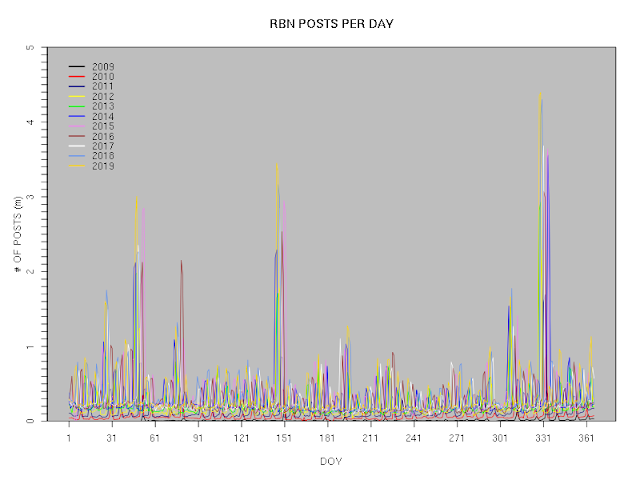## 2020-01-15

### Summary File for RBN data, 2009 to 2019

The complete set of RBN data for 2009 to the end of 2019, after uncompression, is some 80GB in size. As not all analyses need the complete dataset, I have constructed a summary file (rbn-summary-data.xz) that contains an overview of the data and which is sufficient for many kinds of analysis that do not depend on the details of individual posts to the RBN. (The basic script used to generate this summary file may be found here; the actual summary file is created by running the basic script for each individual year from 2009 to 2019 and concatenating the results after removing the header line from all except the first year.)

The summary file, after being uncompressed, comprises a single large table of values separated by white space. The name of each column (there are twelve columns in all) is on the first row. The columns are:
1. band: a string that identifies the band pertaining to this row. Typical values are "15m" or "160m"; if a row contains data that are not distinguished by band, then the characters "NA" are used.
2. mode: a string that identifies the mode pertaining to this row. Typical values are "CW" or "RTTY"; if a row contains data that are not distinguished by mode, then the characters "NA" are used.
3. type: a single character that identifies whether the data on this row are for a period of a year ("A"), a month ("M") or a day ("D").
4. year: the numeric four-digit value of the year to which the current row pertains.
5. month: the numeric value of the month (January = 1, etc.) of the data in this row. If the data are of type A or D, then this element has the value "NA".
6. doy: the numeric value of the day number of the year (January 1st = 1, etc.). The maximum value in each year is 366 (even if the year is not a leap year). In the event that the year is not a leap year, the data in columns 7, 8 and 9 will be set to 0 when doy is 366. If the data are of type A or M, then this element has the value "NA".
7. posts: the total number of posts recorded by the RBN for the band, mode and period identified by the first six columns.
8. calls: the total number of distinguishable calls recorded by the RBN for the band, mode and period identified by the first six columns.
9. posters: the total number of distinguishable posters recorded by the RBN for the band, mode and period identified by the first six columns.
10. scatter: the value of a scatter metric that characterises the geography of the RBN for the band, mode and period identified by the first six columns. The scatter metric is the sum of all possible distance pairs of good posters (measure in km), divided by the number of distance pairs.
11. good posters: the total number of distinguishable posters recorded by the RBN for the band, mode and period identified by the first six columns, and for which location data are available from the RBN.
12.  grid metric: the total number of G(15, 100) grid cells that contain good posters.
For example, the first two lines of the summary file are (presented here as a table, in order to make it easier to view on more devices):

 band NA mode NA type A year 2009 month NA doy NA posts 5007040 calls 143724 posters 151 scatter 5541 good_posters 150 grid_metric 22

This tells us that the first line of actual data in the file comprises annual data for the year 2009, with no separation by band or mode. In 2009, we see that there were 50,007,040 posts of 143,724 callsigns by 151 posters; the scatter metric, which is a measure of the geographic dispersion of the posters on the RBN., was 5,541; 150 different posters contributed the data, spread across 22 distinct G(15, 100) grid cells.

The summary file allows rather rapid analysis of the RBN overview statistics. For example, a plot of the daily number of posts covering the period from the inception of the RBN to the end of 2019 ---- can be generated on an ordinary desktop PC in about five seconds. From this plot, for example, we can immediately see that the largest number of daily posts occurred during the 2019 running of the CQ WW CW contest in late November (the second-highest cluster of peaks is for the CQ WPX contest, and the third is for the ARRL DX CW contest); also, the burst of activity that coincides with weekends is unmistakable.